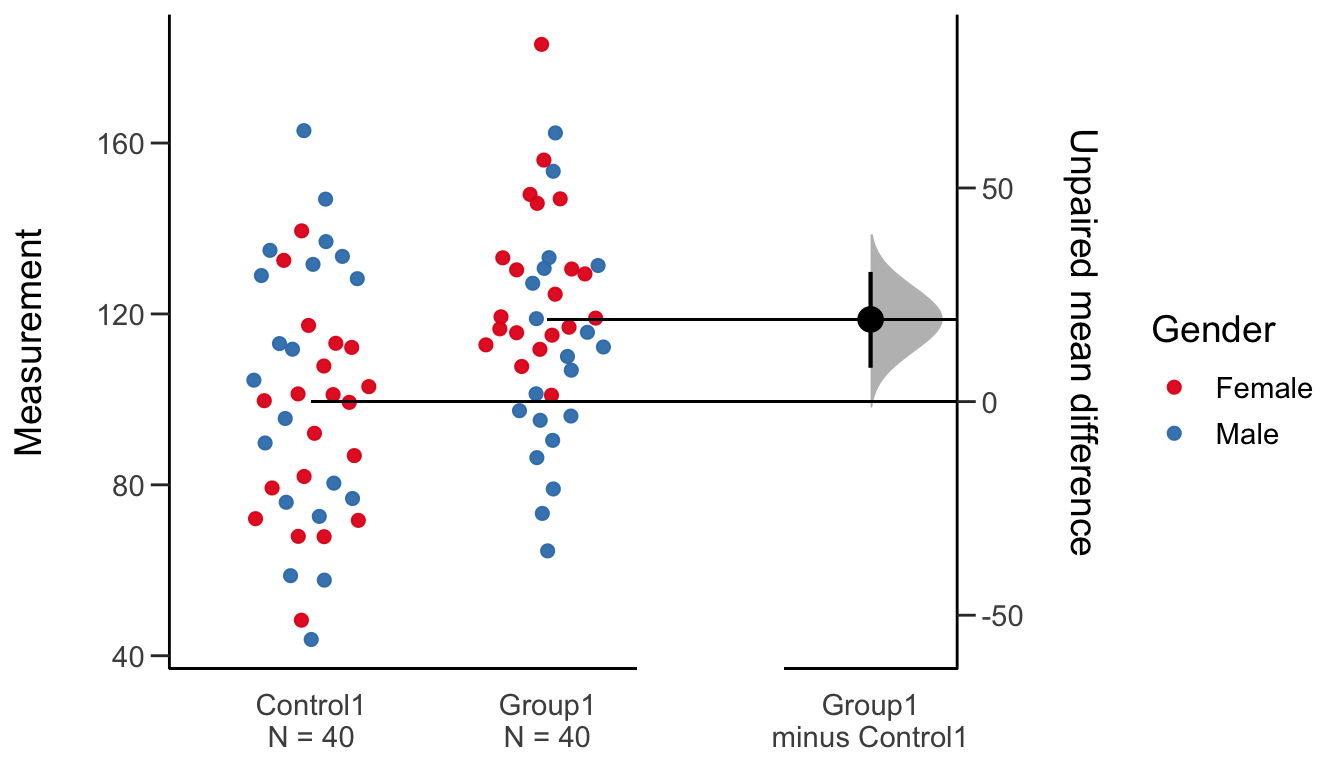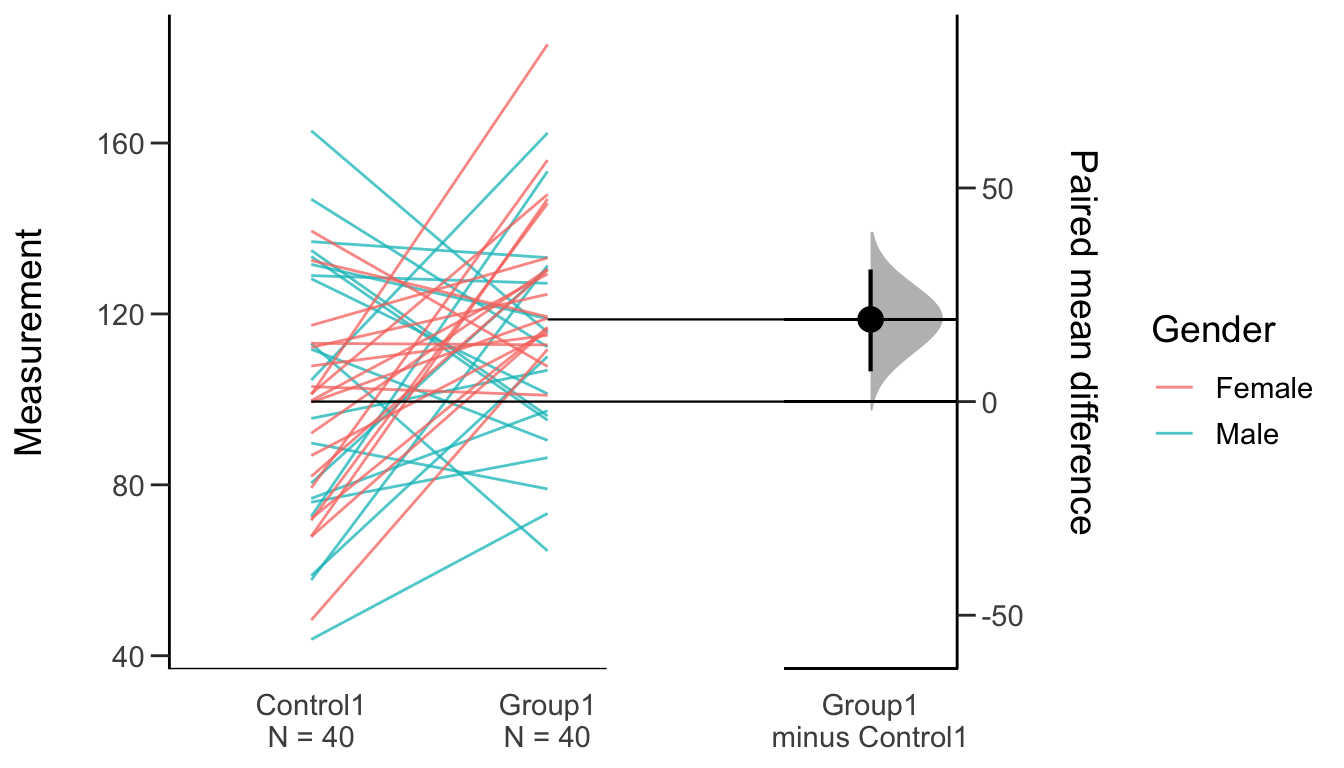# Create Data

For this vignette, we will create and use a synthetic dataset.

``````library(dplyr)

set.seed(54321)

N = 40
c1 <- rnorm(N, mean = 100, sd = 25)
c2 <- rnorm(N, mean = 100, sd = 50)
g1 <- rnorm(N, mean = 120, sd = 25)
g2 <- rnorm(N, mean = 80, sd = 50)
g3 <- rnorm(N, mean = 100, sd = 12)
g4 <- rnorm(N, mean = 100, sd = 50)
gender <- c(rep('Male', N/2), rep('Female', N/2))
id <- 1: N

wide.data <-
tibble::tibble(
Control1 = c1, Control2 = c2,
Group1 = g1, Group2 = g2, Group3 = g3, Group4 = g4,
Gender = gender, ID = id)

my.data   <-
wide.data %>%
tidyr::gather(key = Group, value = Measurement, -ID, -Gender)

``````## # A tibble: 6 x 4
##   Gender    ID Group    Measurement
##   <chr>  <int> <chr>          <dbl>
## 1 Male       1 Control1        95.5
## 2 Male       2 Control1        76.8
## 3 Male       3 Control1        80.4
## 4 Male       4 Control1        58.7
## 5 Male       5 Control1        89.8
## 6 Male       6 Control1        72.6``````

This dataset is a tidy dataset, where each observation (datapoint) is a row, and each variable (or associated metadata) is a column. `dabestr` requires that data be in this form, as do other popular R packages for data visualization and analysis.

# The Gardner-Altman Two Group Estimation Plot

## Unpaired

The `dabest` function is the main workhorse of the `dabestr` package. To create a two-group estimation plot (aka a Gardner-Altman plot), specify:

• the `x` and `y` columns,
• whether the comparison is `paired = TRUE` or `paired = FALSE`,
• and the groups to be compared via `idx`.
``````library(dabestr)

two.group.unpaired <-
my.data %>%
dabest(Group, Measurement,
# The idx below passes "Control" as the control group,
# and "Group1" as the test group. The mean difference
# will be computed as mean(Group1) - mean(Control1).
idx = c("Control1", "Group1"),
paired = FALSE)

# Calling the object automatically prints out a summary.
two.group.unpaired ``````
``````## DABEST (Data Analysis with Bootstrap Estimation) v0.2.0
## =======================================================
##
## Variable: Measurement
##
## Unpaired mean difference of Group1 (n=40) minus Control1 (n=40)
##  19.2 [95CI  7.92; 30.4]
##
##
## 5000 bootstrap resamples.
## All confidence intervals are bias-corrected and accelerated.``````

To create a two-group estimation plot (aka a Gardner-Altman plot), simply use `plot(dabest.object)`.

Advanced R users would be interested to learn that `dabest` produces an object of class `dabest`. There is a generic S3 `plot` method for `dabest` objects that produces the estimation plot.

``plot(two.group.unpaired, color.column = Gender)``This is known as a Gardner-Altman estimation plot, after Martin J. Gardner and Douglas Altman who were the first to publish it in 1986.

The key features of the Gardner-Altman estimation plot are:

1. All data points are plotted.
2. The mean difference (the effect size) and its 95% confidence interval (95% CI) is displayed as a point estimate and vertical bar respectively, on a separate but aligned axes.

The estimation plot produced by `dabest` differs from the one first introduced by Gardner and Altman in one important aspect. `dabest` derives the 95% CI through nonparametric bootstrap resampling. This enables visualization of the confidence interval as a graded sampling distribution.

The 95% CI presented is bias-corrected and accelerated (ie. a BCa bootstrap). You can read more about bootstrap resampling and BCa correction in this vignette.

## Paired

If you have paired or repeated observations, you must specify the `id.col`, a column in the data that indicates the identity of each paired observation. This will produce a Tufte slopegraph instead of a swarmplot.

``````two.group.paired <-
my.data %>%
dabest(Group, Measurement,
idx = c("Control1", "Group1"),
paired = TRUE, id.col = ID)

# The summary indicates this is a paired comparison.
two.group.paired ``````
``````## DABEST (Data Analysis with Bootstrap Estimation) v0.2.0
## =======================================================
##
## Variable: Measurement
##
## Paired mean difference of Group1 (n=40) minus Control1 (n=40)
##  19.2 [95CI  7.08; 30.9]
##
##
## 5000 bootstrap resamples.
## All confidence intervals are bias-corrected and accelerated.``````
``plot(two.group.paired, color.column = Gender)``# The Cummings estimation plot

## Multi-two group

To create a multi-two group plot, one will need to specify a list, with each element of the list corresponding to the each two-group comparison.

``````multi.two.group.unpaired <-
my.data %>%
dabest(Group, Measurement,
idx = list(c("Control1", "Group1"),
c("Control2", "Group2")),
paired = FALSE
)

multi.two.group.unpaired ``````
``````## DABEST (Data Analysis with Bootstrap Estimation) v0.2.0
## =======================================================
##
## Variable: Measurement
##
## Unpaired mean difference of Group1 (n=40) minus Control1 (n=40)
##  19.2 [95CI  7.92; 30.4]
##
## Unpaired mean difference of Group2 (n=40) minus Control2 (n=40)
##  -23.9 [95CI  -45.1; -4.08]
##
##
## 5000 bootstrap resamples.
## All confidence intervals are bias-corrected and accelerated.``````
``plot(multi.two.group.unpaired, color.column = Gender)``